# Complex Analysis : Analytic and Harmonic Functions

## Example Questions

### Example Question #1 : Analytic And Harmonic Functions

Find a Harmonic Conjugate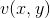of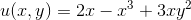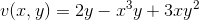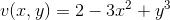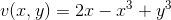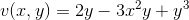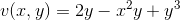Explanation:is said to be a harmonic conjugate of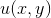if their are both harmonic in their domain and their first order partial derivatives satisfy the Cauchy-Riemann Equations. Computing the partial derivatives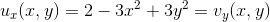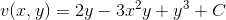whereis any arbitrary constant.

### Example Question #1 : Analytic And Harmonic Functions

Given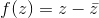, where does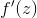exist?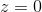NowhereThe Entire Complex Plane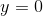Nowhere

Explanation:

Rewriting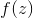in real and complex components, we have that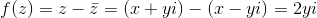So this implies that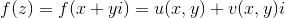where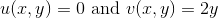Therefore, checking the Cauchy-Riemann Equations, we have that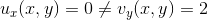So the Cauchy-Riemann equations are never satisfied on the entire complex plane, sois differentiable nowhere.

### All Complex Analysis Resources